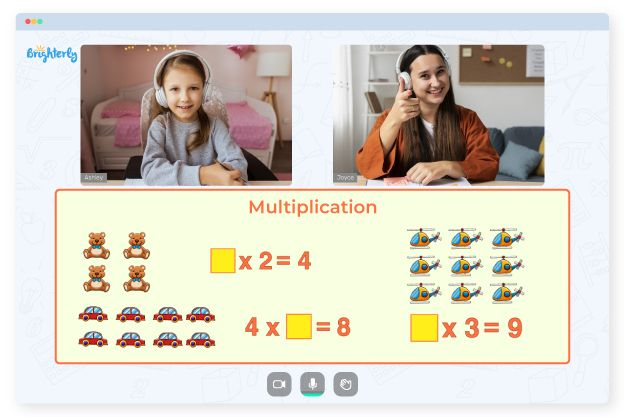# 2 Digit by 1 Digit Multiplication Worksheets

Multiplication can be complex for kids when it gets to two-digit numbers. Their young minds may get things mixed up and multiply from the right instead of the left. To give your child a better shot at understanding this math concept, get 2 digit by 1 digit multiplication worksheets. If you want to know more, this article will discuss the worksheets’ relevance.

## 2-Digit by 1-Digit Multiplication Worksheets from Brighterly

Brighterly has professional tutors that can make your child’s elementary math journey go smoothly. They teach kids how to multiply numbers by using tools like the two-digit by one-digit multiplication worksheets. Students registered at Brighterly get acquainted with terminologies like the area method, box approach, and lattice technique from the worksheets. And the use of these worksheets is a result of the tutors always looking for new ways to help students succeed.

Math for Kids

Is Your Child Struggling With Math?
1:1 Online Math TutoringOne of the fundamental parts of multiplication is multiplying two-digit integers by one. But not every child understands it without help. That is why Brighterly offers a multiplication 2-digit by 1-digit worksheet to help those students catch up. Using free 2-digit by 1-digit multiplication worksheets as PDF files, you can help your child master single-digit multiplication without breaking the bank.### 2 Digit by 1 Digit Multiplication Worksheets PDF

Two Digit By One Digit Multiplication Worksheets### 2 Digit by 1 Digit Multiplication Worksheets PDF

Multiplication 2 Digit By 1 Digit Worksheet### 2 Digit by 1 Digit Multiplication Worksheets PDF

2 Digit By 1 Digit Multiplication Worksheets### 2 Digit by 1 Digit Multiplication Worksheets PDF

Multiplication Worksheets 2 Digit By 1 Digit

## How 2-Digit by 1-Digit Multiplication Worksheets Can Make Learning Exciting

Learning multi-digit multiplication using 2-digit by 1-digit multiplication worksheets in PDF is fun for youngsters. While going through worksheets, your kids can work with the laid-out examples to get step-by-step information on how to tackle the math problem. Multiplication worksheets on 2-digit by 1-digit numbers can have colorful illustrations, riddles, and other fun activities that visualize math problems. Even if kids make a few blunders while tallying up their final results, they will better understand multiplication.

### More Multiplication Worksheets

Need help with Multiplication?• Is your child having trouble mastering multiplication concepts?
• Consider starting lessons with an online tutor.

Does your child need extra assistance with understanding the concept of multiplication? An online tutor could be the answer.